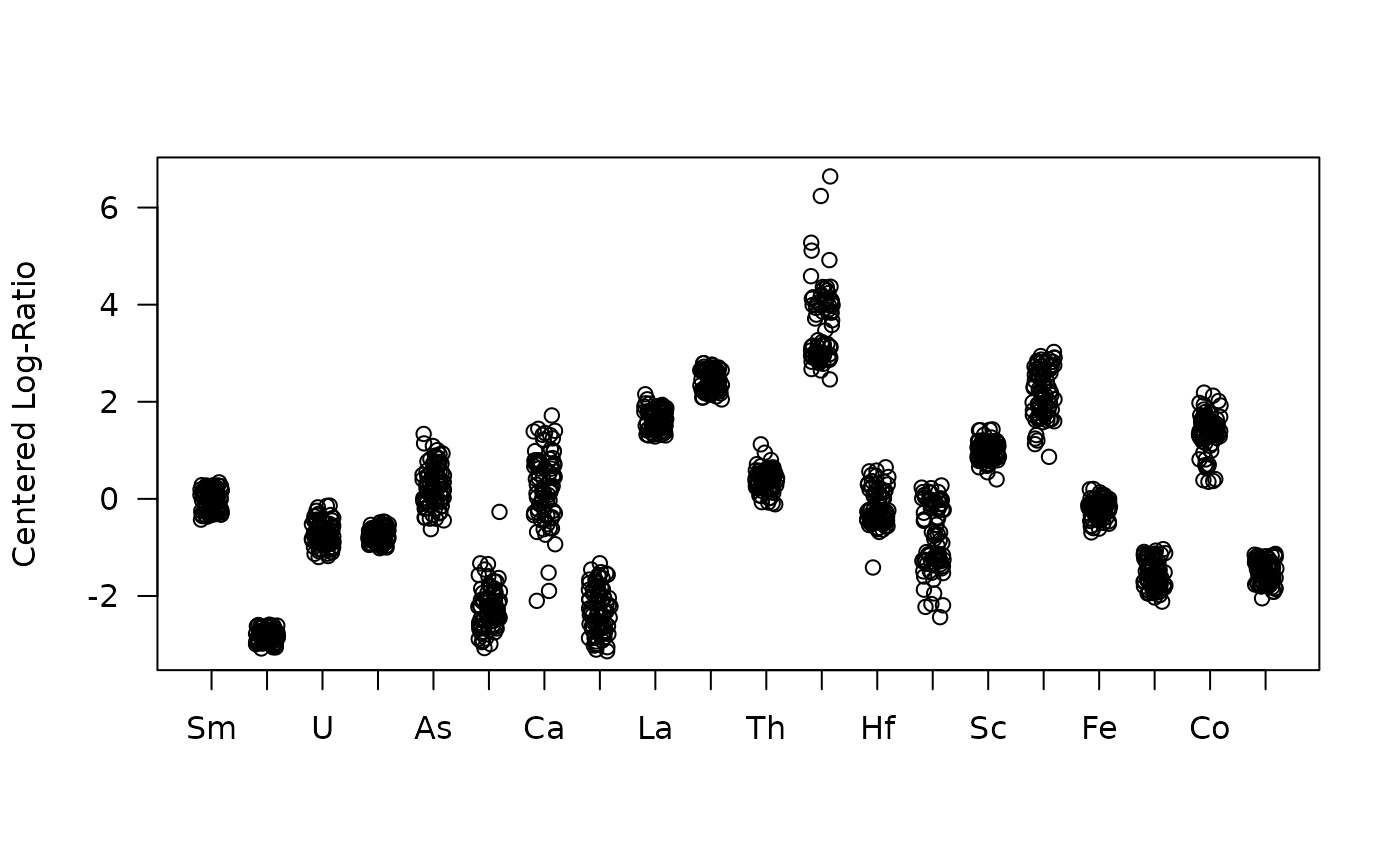Displays a matrix of ternary plots.

## Usage

# S4 method for CompositionMatrix,missing
plot(x, ..., margin = NULL)

## Arguments

x

A CompositionMatrix object.

...

Further graphical parameters.

margin

A character string or an integer giving the index of the column to be used as the third part of the ternary plots. If NULL (the default), marginal compositions will be used (i.e. the geometric mean of the non-selected parts).

## Value

plot() is called for its side-effects: is results in a graphic being displayed (invisibly return x).

isopleuros::ternary_pairs(), isopleuros::ternary_plot()

Other plot methods: as_graph(), barplot(), plot_logratio

N. Frerebeau

## Examples

## Data from Aitchison 1986
data("hongite")

## Coerce to compositional data
coda <- as_composition(hongite)

## Ternary plots
plot(coda)